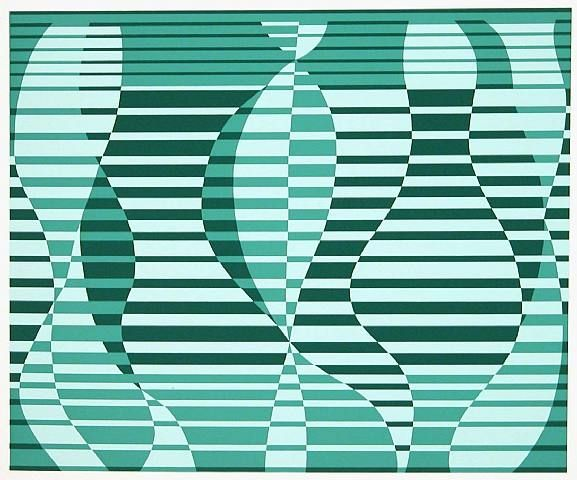Course Title: Ordinary Differential Equations MATH 6410 - 1 Andrejs Treibergs http://www.math.utah.edu/~treiberg/M6416.html M, W, F, 2:00 - 2:50 in JWB 308 10:40-11:30 M, W, F, in JWB 224 (tent.) treiberg@math.utah.edu Math 5210 or consent of instructor. Gerald Teschl, Ordinary Differential Equations and Dynamical Systems A.M.S., 2012 ISBN 978-0-8218-8749-3. List of supplementary materials used in the course.## Math 6410 - 1 Homework

Math 6410 - 1 Slides on the Hartman-Grobman Theorem.

Math 6410 - 1 Fall 2013

In this first semester of a year long graduate course in differential equations, we shall focus on ordinary differential equations and dynamical systems. The second semester, Math 6420 taught by P. Bressloff, will emphasize partial differential equations. In this course, along with the Math 6420, we shall try to cover the syllabus for the qualifying exam in differential equations. Although some mathematical sophistication is required to take the course, we shall provide any background materials needed by the class.

We shall begin by following Teschl's text. We cover existence, uniqueness and continuous dependence of solutions of ordinary differential equations, stability theory, bifurcation theory and periodic solutions. Occasionally we'll refer to more advanced texts. We shall discuss as many applications as we can. Topics include (depending on time):

• Introduction to ODE. Applications. Review of calculus.
• Existence, uniqueness and continuity theorems.
• Linear systems and stability. Liapunov's Method.
• Planar systems. Qualitative theory, Lipunov stability. Limit sets and attractors.
• Bifurcation. Chaos.
• Averaging.
• Invariant manifolds and linearizations. Hartman-Grobman theorem.
• Floquet Theory, periodic solutions and their stability.
• Sturm-Liouville Theory.
• Applications to physical and biological systems. Charged particle, coupled pendula, planetary systems. Extensions to delay equations, integro-differential equations. Semigroup approach.

Last updated: 7 / 26 / 16